Win up to 100% scholarship on Aakash BYJU'S JEE/NEET courses with ABNAT Win up to 100% scholarship on Aakash BYJU'S JEE/NEET courses with ABNAT

# JEE Advanced Question Paper 2022 Maths Paper 2

## JEE Advanced 2022 Maths Question Paper 2 with Solutions

SECTION – 1 (Maximum marks : 24)

∙ This section contains EIGHT (08) questions.

∙ The answer to each question is a SINGLE DIGIT INTEGER ranging from 0 TO 9, BOTH INCLUSIVE.

∙ For each question, enter the correct integer corresponding to the answer using the mouse and the on-screen virtual numeric keypad in the place designated to enter the answer.

∙ Answer to each question will be evaluated according to the following marking scheme:

Full Marks : +3 If ONLY the correct integer is entered;

Zero Marks : 0 If the question is unanswered;

Negative Marks : −1 In all other cases.

1. Let α and β be real numbers such that

$$\begin{array}{l}-\frac{\pi}{4}<\beta<0<\alpha<\frac{\pi}{4}.\end{array}$$
If sin(α + β) = ⅓ and cos(α – β) = ⅔, then the greatest integer less than or equal to
$$\begin{array}{l}{\left( {\frac{{\sin \alpha }}{{\cos \beta }} + \frac{{\cos \beta }}{{\sin \alpha }} + \frac{{\cos \alpha }}{{\sin \beta }} + \frac{{\sin \beta }}{{\cos \alpha }}} \right)^2}\end{array}$$
is _______.

Sol.

$$\begin{array}{l}{\left( {\frac{{\sin \alpha }}{{\cos \beta }} + \frac{{\cos \alpha }}{{\sin \beta }} + \frac{{\cos \beta }}{{\sin \alpha }} + \frac{{\sin \beta }}{{\cos \alpha }}} \right)^2}\end{array}$$
$$\begin{array}{l} ={\left( {\frac{{\cos (\alpha-\beta )}}{{\sin \beta \cos \beta }} + \frac{{\cos (\alpha-\beta )}}{{\sin \alpha\cdot \cos \alpha }}} \right)^2}\end{array}$$
$$\begin{array}{l} = {\left( {\frac{4}{3}\left\{ {\frac{1}{{\sin 2\beta }} + \frac{1}{{\sin 2\alpha }}} \right\}} \right)^2}\end{array}$$
$$\begin{array}{l} = \frac{{16}}{9}{\left( {\frac{{2\sin (\alpha+\beta ) \cdot \cos (\alpha-\beta )}}{{\sin 2\alpha\cdot \sin 2\beta }}} \right)^2}\end{array}$$
$$\begin{array}{l} =\frac{{16}}{9}{\left( {\frac{{4 \cdot \frac{1}{3}\cdot \frac{2}{3}}}{{\cos (2\alpha-2\beta )-\cos (2\alpha+ 2\beta)}}} \right)^2}\end{array}$$
$$\begin{array}{l} =\frac{16}{9}\left(\frac{\frac{8}{9}}{2\ cos^2(\alpha-\beta)-1-1+2\ sin^2(\alpha+\beta)}\right)^2\end{array}$$
$$\begin{array}{l} = \frac{{16}}{9}{\left( {\frac{{\frac{8}{9}}}{{\frac{8}{9} – 2 + \frac{2}{9}}}} \right)^2}\end{array}$$
$$\begin{array}{l} = \frac{16}{9}\end{array}$$

= 1

2. If y(x) is the solution of the differential equation xdy – (y2 – 4y)dx = 0 for x > 0, y(1) = 2, and the slope of the curve y = y(x) is never zero, then the value of 10y(√2) is ______.

Sol.

$$\begin{array}{l}\frac{{dy}}{{{y^2} – 4y}} = \frac{{dx}}{x}\end{array}$$
$$\begin{array}{l} \Rightarrow \,\,\,\,\,\frac{1}{4}\left( {\frac{1}{{y – 4}} – \frac{1}{y}} \right)dy = \frac{{dx}}{x}\end{array}$$

Integrating both sides

$$\begin{array}{l}\frac{1}{4}\ln \left| {\frac{{y – 4}}{y}} \right| = \ln \left| x \right| + c\end{array}$$

As, y(1) = 2, c = 0

so,

$$\begin{array}{l}\frac{{y – 4}}{y}=\pm {x^4}\end{array}$$

or,

$$\begin{array}{l}y = \frac{4}{{1 \pm {x^4}}}\end{array}$$

Considering

$$\begin{array}{l}y = \frac{4}{{1 + {x^4}}}\end{array}$$
$$\begin{array}{l}y\left( {\sqrt 2 } \right) = \frac{4}{5}\end{array}$$

So,

$$\begin{array}{l}10y\left( {\sqrt 2 } \right) = 8\end{array}$$

3. The greatest integer less than or equal to

$$\begin{array}{l}\int_1^2 {{{\log }_2}({x^3} + 1)dx + } \int_1^{{{\log }_2}9} {{{({2^x} – 1)}^{\frac{1}{3}}}dx} \end{array}$$
is ______.

Sol.

$$\begin{array}{l}I = \int_1^2 {{{\log }_2}({x^3} + 1)dx}+\int_1^{{{\log }_2}9} {{{({2^x} – 1)}^{\frac{1}{3}}}dx}\end{array}$$

Let

$$\begin{array}{l}\int_1^{{{\log }_2}9} {{{({2^x} – 1)}^{\frac{1}{3}}}dx = {I_2}} \end{array}$$

Let 2x – 1 = t3

⇒ 2xln2dx = 3t2dt

or

$$\begin{array}{l}dx = \frac{{3{t^2}}}{{\ln 2({t^3} + 1)}}dt\end{array}$$

So,

$$\begin{array}{l}I=\int_1^2 {{{\log }_2}({x^3} + 1)dx}+\int_1^2 {\frac{{3{t^3}}}{{\ln 2({t^3} + 1)}}dt} \end{array}$$

or

$$\begin{array}{l}I = \int_1^2 {\left( {{{\log }_2}({t^3} + 1) + t \cdot \frac{{3{t^2}}}{{({t^3} + 1)\ln 2}}} \right)dt} \end{array}$$
$$\begin{array}{l}=\left. {t \cdot {{\log }_2}({t^3} + 1)} \right|_1^2\end{array}$$

= 2log2 9 – 1log22

= 2log2 9 – 1

So, [I] = 5

4. The product of all positive real values of x satisfying the equation

$$\begin{array}{l}x^{(16(\log_5 x)^3-68\log_5x)}=5^{-16}\end{array}$$
is _____.

Sol. Taking log to the base 5 on both sides

(16(log5x)3 – 68 (log5x)) (log5x) = –16

Let (log5x) = t

16t4 – 68t2 + 16 = 0

OR 4t4 – 16t2t2 + 4 = 0

OR (4t2 – 1) (t2 – 4) = 0

OR

$$\begin{array}{l}t =\pm \frac{1}{2},\ \pm 2\end{array}$$

So

$$\begin{array}{l}{\log _5}x = \pm \frac{1}{2}{\rm{ OR }} \pm {\rm{2}}\end{array}$$
$$\begin{array}{l} \Rightarrow x = {5^{\frac{1}{2}}},\,\,\,\,{5^{\frac{{ – 1}}{2}}},\,\,\,\,{5^2},\,\,{5^{ – 2}}\end{array}$$

5. If

$$\begin{array}{l}\beta = \mathop {\lim }\limits_{x \to 0} \frac{{{e^{{x^3}}} – {{(1 – {x^3})}^{\frac{1}{3}}} + ({{(1 – {x^2})}^{\frac{1}{2}}} – 1)\sin x}}{{x{{\sin }^2}x}},\end{array}$$
then the value of 6β is _____.

Sol.

$$\begin{array}{l}\beta=\displaystyle \lim_{x \to 0}\frac{e^{x^3}-(1-x^3)^\frac{1}{3}+((1-x^2)^\frac{1}{2}-1)sin x}{x\cdot \frac{sin^2 x}{x^2}\cdot x^2}\end{array}$$
$$\begin{array}{l}\beta= \displaystyle \lim_{x \to 0}\left ( \frac{\left ( 1+x^3+\frac{x^6}{2!}+\cdots \right )-\left ( 1-\frac{1}{3}x^3+\left ( \frac{-1}{9} \right )x^6+\cdots \right )+\left ( -\frac{1}{2}x^2-\frac{1}{8}x^4+\cdots \right )\left ( x-\frac{x^3}{3!}+\cdots \right )}{x^3} \right )\end{array}$$
$$\begin{array}{l}\beta=\displaystyle \lim_{x \to 0} \frac{x^3\left( 1 + \frac{1}{3} – \frac{1}{2}\right)}{x^3}\end{array}$$
(Neglecting higher powers of x)

So,

$$\begin{array}{l}\beta = \frac{5}{6}\end{array}$$

or 6β = 5

6. Let β be a real number. Consider the matrix

$$\begin{array}{l}A=\begin{pmatrix}\beta & 0 & 1\\2 & 1 & -2\\3 & 1 & -2\\\end{pmatrix}\end{array}$$
. If A7 – (β – 1)A6 – βA5 is a singular matrix, then the value of 9β is _____.

Sol.

$$\begin{array}{l}A = \left( {\begin{array}{*{20}{c}}\beta &0&1\\2&1&{ – 2}\\3&1&{ – 2}\end{array}} \right)\end{array}$$

det. (A) = – 1 …(1)

For A7 – (β – 1)A6 – βA5 to be singular

|A5| |A2 – (β – 1)A – β| = 0

⇒ |A5| |(A + I) (A – βI)| = 0 …(2)

∴ |A5| |A + I| |A – βI| = 0

As |A| ≠ 0

|A + I| or |A – βI| = 0

$$\begin{array}{l} \Rightarrow \begin{pmatrix}\beta+1 & 0 & 1 \\2 & 2 & -2 \\3 & 1 & -1 \\\end{pmatrix}=0\ \left\{|A+I|\ne 0 \right\}\end{array}$$

Given, – 1 = 0 (Rejected)

$$\begin{array}{l} \therefore \left| {A – \beta I} \right| = \left| {\begin{array}{*{20}{c}}0&0&1\\2&{1 – \beta }&{ – 2}\\3&1&{ – 2 – \beta }\end{array}} \right| = 0\end{array}$$

⇒ 2 – 3(1 – β) = 0

⇒ 2 – 3 + 3β = 0

$$\begin{array}{l} \Rightarrow \beta = \frac{1}{3}\end{array}$$

∴ 9β = 3

7. Consider the hyperbola

$$\begin{array}{l}\frac{{{x^2}}}{{100}} – \frac{{{y^2}}}{{64}} = 1\end{array}$$
with foci at S and S1, where S lies on the positive x-axis. Let P be a point on the hyperbola, in the first quadrant. Let ∠SPS1 = α, with α < π/2. The straight line passing through the point S and having the same slope as that of the tangent at P to the hyperbola, intersects the straight line S1P at P1. Let δ be the distance of P from the straight line SP1, and β = S1P. Then the greatest integer less than or equal to
$$\begin{array}{l}\frac{{\beta \delta }}{9}\sin \frac{\alpha }{2}\end{array}$$
is ______.

Sol.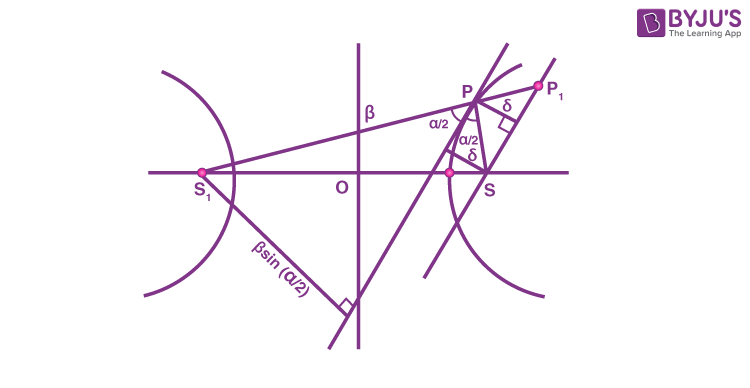Product of distances of any tangent from two foci = b2

$$\begin{array}{l}\delta\times \beta \sin \frac{\alpha }{2} = {b^2}\end{array}$$

So,

$$\begin{array}{l}\frac{{\beta \delta \sin \frac{\alpha }{2}}}{9} = \frac{{{b^2}}}{9} = \frac{{64}}{9}\end{array}$$

Greatest integer less than or equal to

$$\begin{array}{l}\frac{{\beta \delta \sin \frac{\alpha }{2}}}{9}\ \text{is}\ 7.\end{array}$$

8. Consider the functions f, g : ℝ → ℝ defined by

$$\begin{array}{l}f(x) = {x^2} + \frac{5}{{12}}\ \text{and}\ g(x) = \left\{ {\begin{array}{*{20}{c}}{2\left( {1 – \frac{{4|x|}}{3}} \right)}&,&{\left| x \right| \le \frac{3}{4},}\\0&,&{\left| x \right| > \frac{3}{4}.}\end{array}} \right.\end{array}$$
If α is the area of the region {(x, y)∈ ℝ × ℝ: |x| ≤ ¾, 0 ≤ y ≤ min{f(x), g(x)}},, then the value of 9α is ______.

Sol. Figure can be drawn as shown,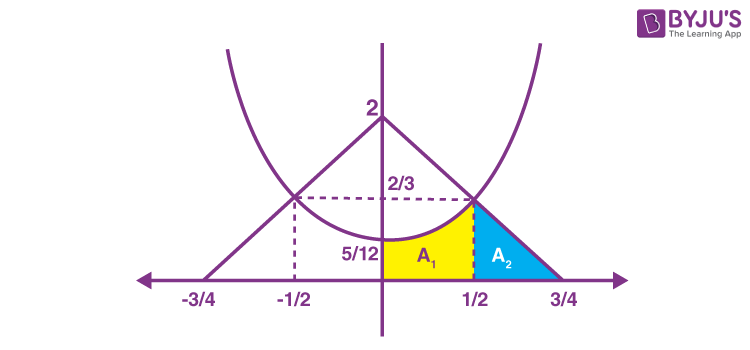Required area = 2·(A1 + A2)

$$\begin{array}{l} \Rightarrow \alpha=\left( {\int\limits_0^{1/2} {\left( {{x^2} + \frac{5}{{12}}} \right)dx + \frac{1}{2}\left( {\frac{3}{4} – \frac{1}{2}} \right) \cdot \frac{2}{3}} } \right) \times 2\end{array}$$
$$\begin{array}{l} \Rightarrow \alpha=\left({\left.{\left({\frac{{x^3}}{3}+\frac{5x}{12}} \right)} \right|_0^{1/2}+\frac{1}{2}\cdot \frac{1}{4}\cdot \frac{2}{3}}\right) \times 2\end{array}$$
$$\begin{array}{l} \Rightarrow \alpha = \left( {\frac{1}{4} + \frac{1}{{12}}} \right) \times 2\end{array}$$
$$\begin{array}{l} \Rightarrow \alpha = \frac{2}{3}\end{array}$$

⇒ 9α = 6

SECTION – 2 (Maximum marks : 24)

This section contains SIX (06) questions.

Each question has FOUR options (A), (B), (C) and (D). ONE OR MORE THAN ONE of these four option(s) is (are) correct answer(s).

∙ For each question, choose the option(s) corresponding to (all) the correct answer(s).

∙ Answer to each question will be evaluated according to the following marking scheme:

Full Marks : +4 ONLY if (all) the correct option(s) is(are) chosen;

Partial Marks : +3 If all the four options are correct but ONLY three options are chosen;

Partial Marks : + 2 If three or more options are correct but ONLY two options are chosen, both of which are correct;

Partial Marks : +1 If two or more options are correct but ONLY one option is chosen and it is a correct option;

Zero Marks : 0 If none of the options is chosen (i.e. the question is unanswered);

Negative Marks : –2 In all other cases.

9. Let PQRS be a quadrilateral in a plane, where QR = 1, ∠PQR = ∠QRS = 70°, ∠PQS = 15° and ∠PRS = 40°. If ∠RPS = θ°, PQ = α and PS = β, then the interval(s) that contain(s) the value of 4αβ sinθ° is/are

(A) (0, √2)

(B) (1, 2)

(C) (√2, 3)

(D) (2√2, 3√2)

Sol. Figure as shown, can be drawn on the basis of given data.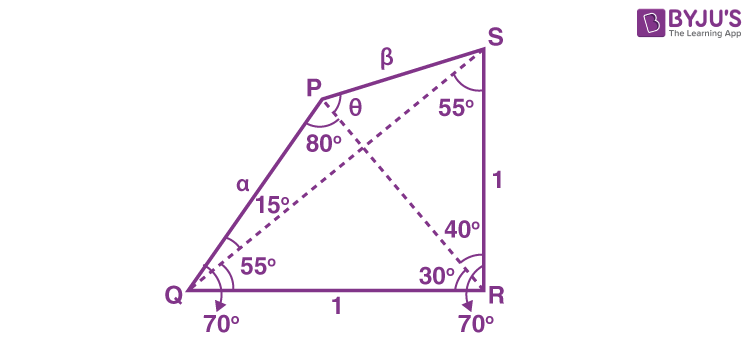Applying sine rule in ΔPQR,

$$\begin{array}{l}\frac{\alpha }{{\sin 30^\circ }} = \frac{1}{{\sin 80^\circ }}\end{array}$$
$$\begin{array}{l}\Rightarrow \alpha=\frac{1}{2\sin 80^\circ}\cdots (i)\end{array}$$

Applying sine rule in ΔPRS,

$$\begin{array}{l}\frac{\beta }{{\sin 40^\circ }} = \frac{1}{{\sin \theta }}\end{array}$$

⇒ βsinθ = sin40° … (ii)

From (i) & (ii),

$$\begin{array}{l}4\alpha \beta \sin \theta=4\cdot\frac{1}{{2\sin 80^\circ }}\cdot\sin 40^\circ=\frac{1}{{\cos 40^\circ }}\end{array}$$

Using cos 0° = 1, cos 30° = √3/2, cos 45° = 1/√2, and cos 60° = 1/2

Only Options (A) and (B) are correct.

10. Let

$$\begin{array}{l}\alpha=\sum_{k = 1}^\infty \sin^{2k}\left(\frac{\pi}{6}\right)\end{array}$$

Let g ∶ [0, 1] → ℝ be the function defined by

g(x) = 2αx + 2α(1– x)

Then, which of the following statements is/are TRUE?

(A) The minimum value of g(x) is 27/6

(B) The maximum value of g(x) is 1 + 21/3

(C) The function g(x) attains its maximum at more than one point

(D) The function g(x) attains its minimum at more than one point

Sol.

$$\begin{array}{l}\alpha={\sum\limits_{k = 1}^\infty{\left( {\frac{1}{2}} \right)} ^{2k}}=\sum\limits_{k = 1}^\infty{{{\left( {\frac{1}{4}} \right)}^k}=\frac{{{\rm{1/4}}}}{{1-\frac{1}{4}}}}=\frac{1}{3}\end{array}$$

Hence, g(x) = 2x/3 + 2(1 – x)/3

Now,

$$\begin{array}{l}g'(x) = \frac{{\ln 2}}{3}\frac{{({2^{2x/3}}-{2^{1/3}})}}{{{2^{x/3}}}}\end{array}$$

g’(x) = 0 at x = 1/2

And, derivative changes sign from negative to positive at x = 1/2, hence x = 1/2 is point of local minimum as well as absolute minimum of g(x) for x ∈ [0, 1]

Hence, minimum value of g(x) = g(½)

= 21/6 + 21/6 = 27/6

⇒ Option (A) is correct

Maximum value of g(x) is either equal to g(0) or g(1).

g(0) = 1 + 21/3

g(1) = 21/3 +1

Hence (B) and (C) are also correct.

11. Let

$$\begin{array}{l}\bar{z}\ \text{denote the complex conjugate of a complex number z}.\end{array}$$
If z is a non-zero complex number for which both real and imaginary parts of
$$\begin{array}{l}{(\bar z)^2} + \frac{1}{{{z^2}}}\end{array}$$
are integers, then which of the following is/are possible value(s) of |z|?

$$\begin{array}{l}(A)\ {\left( {\frac{{43 + 3\sqrt {205} }}{2}} \right)^{1/4}}\end{array}$$
$$\begin{array}{l}(B)\ {\left( {\frac{{7 + \sqrt {33} }}{4}} \right)^{1/4}}\end{array}$$
$$\begin{array}{l}(C)\ {\left( {\frac{{9 + \sqrt {65} }}{4}} \right)^{1/4}}\end{array}$$
$$\begin{array}{l}(D)\ {\left( {\frac{{7 + \sqrt {13} }}{6}} \right)^{1/4}}\end{array}$$

Sol. Let z = r.eiθ

So,

$$\begin{array}{l}{(\bar z)^2} + \frac{1}{{{z^2}}} = \left( {{r^2} + \frac{1}{{{r^2}}}} \right){e^{-2i\theta }} = a + ib\,\,{\rm{(say)}}\end{array}$$
, Where a, bZ

So,

$$\begin{array}{l}{\left( {{r^2} + \frac{1}{{{r^2}}}} \right)^2} = {a^2} + {b^2}\end{array}$$

r8 – (a2 + b2 – 2)r4 + 1 = 0

$$\begin{array}{l} \Rightarrow {r^4}=\frac{{({a^2} + {b^2}-2)}\pm \sqrt{(a^2 +b^2-2)^2-4}}{2}\end{array}$$

For a2 + b2 = 45 (i.e (a, b) = (± 6, ±3) or (±3, ±6)

We get

$$\begin{array}{l}r = {\left( {\frac{{43 + 3\sqrt {205} }}{2}} \right)^{1/4}}\end{array}$$

12. Let G be a circle of radius R > 0. Let G1, G2, …, Gn be n circles of equal radius r > 0. Suppose each of the n circles G1, G2, …, Gn touches the circle G externally. Also, for i = 1, 2, …, n − 1, the circle Gi touches Gi+1 externally, and Gn touches G1 externally. Then, which of the following statements is/are TRUE ?

(A) If n = 4, then (√2 – 1)r < R

(B) If n = 5, then r < R

(C) If n = 8, then (√2 – 1)r < R

(D) If n = 12, then √2(√3 + 1)r > R

Sol. Refer to diagram,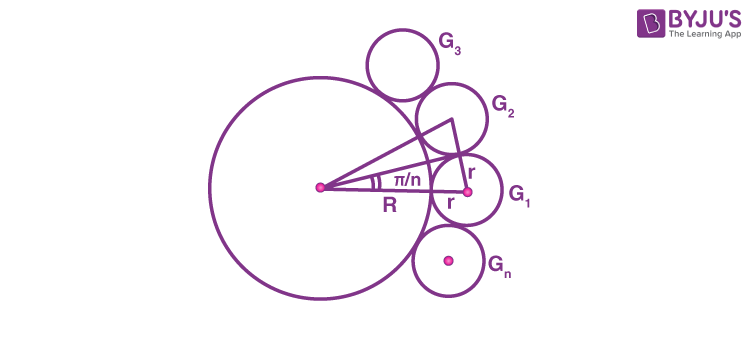$$\begin{array}{l}\sin \left( {\frac{\pi }{n}} \right) = \frac{r}{{R + r}}\end{array}$$
$$\begin{array}{l} \Rightarrow \frac{R}{r} + 1 = {\rm{cosec}}\left( {\frac{\pi }{n}} \right)\end{array}$$
$$\begin{array}{l}\Rightarrow R = r\left[ {{\rm{cosec}}\left( {\frac{\pi }{n}} \right)-1} \right]\end{array}$$

(A) n = 4, R = r(√2 – 1)

(B)

$$\begin{array}{l}n = 5,\,\,R = r\left( {{\rm{cosec}}\frac{\pi }{5}-1} \right)\end{array}$$
$$\begin{array}{l} \Rightarrow R < r\left( {{\rm{cosec}}\frac{\pi }{6}-1} \right) \Rightarrow \,\,R < r\end{array}$$

(C)

$$\begin{array}{l}n = 8,\,\,R = r\left[ {{\rm{cosec}}\left( {\frac{\pi }{8}} \right)-1} \right]\end{array}$$
$$\begin{array}{l} \Rightarrow R > r\left( {{\rm{cosec}}\left( {\frac{\pi }{4}} \right)-1} \right)\end{array}$$
$$\begin{array}{l} \Rightarrow R > r\left( {\sqrt 2 -1} \right)\end{array}$$

(D)

$$\begin{array}{l}n = 12,\,\,R = r\left[ {{\rm{cosec}}\left( {\frac{\pi }{{12}}} \right)-1} \right]\end{array}$$
$$\begin{array}{l} \Rightarrow R=\left[{\sqrt 2\left( {\sqrt3+ 1}\right)-1}\right]r\end{array}$$
$$\begin{array}{l} \Rightarrow R <\sqrt 2\left({\sqrt 3+1} \right)r\end{array}$$

13. Let

$$\begin{array}{l}\hat{i},\ \hat{j},\ \text{and}\ \hat{k}\end{array}$$
be the unit vectors along the three positive coordinate axes. Let
$$\begin{array}{l}\vec{a}=3\hat{i}+\hat{j}-\hat{k}\\\vec{b}=\hat{i}+b_2\hat{j}+b_3\hat{k},\ b_2,b_3\in \mathbb{R}\\\vec{c}=c_1\hat{i}+c_2\hat{j}+c_3\hat{k},\ c_1,c_2,c_3\in \mathbb{R}\end{array}$$

be three vectors such that b2b3 > 0,

$$\begin{array}{l}\vec a \cdot \vec b = 0\end{array}$$
and

$$\begin{array}{l}\left( {\begin{array}{*{20}{c}}0&{ – {c_3}}&{{c_2}}\\{{c_3}}&0&{ – {c_1}}\\{ – {c_2}}&{{c_1}}&0\end{array}} \right)\left( {\begin{array}{*{20}{c}}1\\{{b_2}}\\{{b_3}}\end{array}} \right) = \left( {\begin{array}{*{20}{c}}{3 – {c_1}}\\{1 – {c_2}}\\{ – 1 – {c_3}}\end{array}} \right)\end{array}$$
.

Then, which of the following is/are TRUE?

$$\begin{array}{l}(A)\ \vec a \cdot \vec c = 0\end{array}$$
$$\begin{array}{l}(B)\ \vec b \cdot \vec c – = 0\end{array}$$
$$\begin{array}{l}(C)\ \left| {\vec b} \right| > \sqrt {10} \end{array}$$
$$\begin{array}{l}(D)\ \left| {\vec c} \right| \le \sqrt {11} \end{array}$$

Sol.

$$\begin{array}{l}\left[ {\hat i\,\hat j\,\hat k} \right]\left[ {\begin{array}{*{20}{c}}0&{ – {c_3}}&{{c_2}}\\{{c_3}}&0&{ – {c_1}}\\{ – {c_2}}&{{c_1}}&0\end{array}} \right]\left[ {\begin{array}{*{20}{c}}1\\{{b_2}}\\{{b_3}}\end{array}} \right] = \left[ {\hat i\,\hat j\,\hat k} \right]\left[ {\begin{array}{*{20}{c}}{3 – {c_1}}\\{1 – {c_2}}\\{ – 1 – {c_3}}\end{array}} \right]\end{array}$$
$$\begin{array}{l} \Rightarrow \vec{c}\times \vec{b}=\vec a – \vec c \cdots(i)\end{array}$$
$$\begin{array}{l} \Rightarrow \left( {\vec c \times \vec b} \right) \cdot \vec b = \vec a \cdot \vec b – \vec c \cdot \vec b = 0\end{array}$$
$$\begin{array}{l} \Rightarrow \vec b \cdot \vec c = 0\end{array}$$

Again from (i)

$$\begin{array}{l}\left( {\vec c \times \vec b} \right) \cdot \vec c = \vec a \cdot \vec c – {\left| {\vec c} \right|^2} = 0\end{array}$$
$$\begin{array}{l} \Rightarrow {\left|{\vec c}\right|^2}=\left|{\vec a}\right|\left| {\vec c} \right|\cos\theta\ \text{where}\ \theta=\vec a ^\wedge \vec c\end{array}$$
$$\begin{array}{l} \Rightarrow \left| {\vec c} \right| \ge \left| {\vec a} \right| \Rightarrow \left| {\vec c} \right| \geq \sqrt {11}\end{array}$$

Given that

$$\begin{array}{l}\vec a \cdot \vec b = 0\,\,\, \Rightarrow \,\,{b_2} – {b_3} + 3 = 0\end{array}$$

b3b2 = 3

Also b2 · b3 > 0

Now

$$\begin{array}{l}{\left| {\vec b} \right|^2} = 1 + b_2^2 + b_3^2\end{array}$$

= 1 + (b3b2)2 + 2b2b3

= 10 + 2b2b3

$$\begin{array}{l} \Rightarrow {\left| {\vec b} \right|^2} > 10 \Rightarrow \left| {\vec b} \right| > \sqrt {10} \end{array}$$

14. For x ∈ ℝ, let the function y(x) be the solution of the differential equation

$$\begin{array}{l}\frac{{dy}}{{dx}} + 12y = \cos \left( {\frac{\pi }{{12}}x} \right),\,\,\,y\left( 0 \right) = 0.\end{array}$$

Then, which of the following statements is/are TRUE?

(A) y(x) is an increasing function

(B) y(x) is a decreasing function

(C) There exists a real number β such that the line y = β intersects the curve y = y(x) at infinitely many points

(D) y(x) is a periodic function

Sol.

$$\begin{array}{l}\frac{{dy}}{{dx}} + 12y = \cos \left( {\frac{{\pi x}}{{12}}} \right)\end{array}$$
$$\begin{array}{l}I.F. = {e^{12x}} \Rightarrow y.{e^{12x}} = \int\limits_{}^{} {{e^{12x}} \cdot \cos \left( {\frac{{\pi x}}{{12}}} \right)dx + C} \end{array}$$
$$\begin{array}{l} \Rightarrow y\cdot e^{12x}=\frac{e^{12x}}{12^2 + \left(\frac{\pi}{12}\right )^2}\left[12\ cos\frac{\pi x}{12}+\frac{\pi}{12}sin\left(\frac{\pi x}{12}\right) \right]+C\end{array}$$
$$\begin{array}{l}\because y(0)=0\Rightarrow C = -\frac{12}{12^2+\left ( \frac{\pi}{12} \right )^2}\end{array}$$

So

$$\begin{array}{l}y = \frac{1}{\lambda }\left[ {\underbrace {12\cos \left( {\frac{{\pi x}}{{12}}} \right) + \frac{\pi }{{12}}\sin \left( {\frac{{\pi x}}{{12}}} \right)}_{{f_1}\left( x \right)} – 12{e^{ – 12x}}} \right]\end{array}$$
$$\begin{array}{l}\frac{{dy}}{{dx}}=\frac{1}{\lambda }\left[ {\underbrace { – \pi \sin \left( {\frac{\pi x}{12}} \right) + \frac{\pi^2}{12^2}\cos \left( {\frac{{\pi x}}{12}} \right)}_{{f_2}\left( x \right)}+12{e^{-12x}}} \right]\end{array}$$

When x is large then 12e–12x tends to zero.

But f2(x) varies in

$$\begin{array}{l}\left[ {-\sqrt {{\pi ^2} + {{\left(\frac{\pi}{12} \right)}^4}},\ \sqrt{\pi ^2+{\left(\frac{\pi}{12}\right)^4}}}\right]\end{array}$$

Hence dy/dx is changing its sign.

So y(x) is non monotonic for all real number.

Also when x is very large then again – 12e–12x is almost zero but f1(x) is periodic, so there exist some β for which y = β intersect y = y(x) at infinitely many points.

SECTION – 3 (Maximum marks : 12)

∙ This section contains FOUR (04) questions.

∙ Each question has FOUR options (A), (B), (C) and (D). ONLY ONE of these four options is the correct answer.

∙ For each question, choose the option corresponding to the correct answer.

∙ Answer to each question will be evaluated according to the following marking scheme:

Full Marks : +3 If ONLY the correct option is chosen;

Zero Marks : 0 If none of the options is chosen (i.e. the question is unanswered);

Negative Marks : −1 In all other cases.

15. Consider 4 boxes, where each box contains 3 red balls and 2 blue balls. Assume that all 20 balls are distinct. In how many different ways can 10 balls be chosen from these 4 boxes so that from each box at least one red ball and one blue ball are chosen?

(A) 21816

(B) 85536

(C) 12096

(D) 156816

Sol.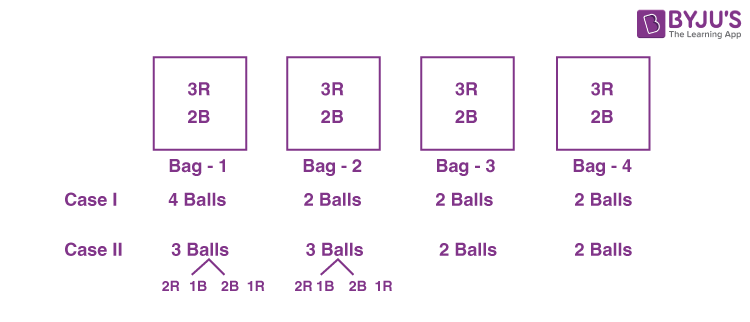4C1 [3 Red 1 Blue 2 Red 2 Blue] + Case II.

$$\begin{array}{l}=^4{C_1}\left[ {^2{C_1}{ + ^3}{C_1}} \right]{\left( {^3{C_1}{\,^2}{C_1}} \right)^3}{ + ^4}{C_2}{\left[ {^3{C_2}{\,^2}{C_1}{ + ^3}{C_1}{\,^2}{C_2}} \right]^2}{\left[ {^3{C_1}{\,^2}{C_1}} \right]^2}\end{array}$$
$$\begin{array}{l}=4\left( 5 \right){\left( 6 \right)^3} + 6{\left( {3 \times 2 + 3} \right)^2}{\left( 6 \right)^2}\end{array}$$

= 4320 + 17496

= 21816 (Option A)

16. If

$$\begin{array}{l}M=\begin{pmatrix}\frac{5}{2} & \frac{3}{2} \\-\frac{3}{2} & -\frac{1}{2} \\\end{pmatrix}\end{array}$$
, then which of the following matrices is equal to M2022?

(A)

$$\begin{array}{l}\left( {\begin{array}{*{20}{c}}{3034}&{3033}\\{ – 3033}&{ – 3032}\end{array}} \right)\end{array}$$

(B)

$$\begin{array}{l}\left( {\begin{array}{*{20}{c}}{3034}&{-3033}\\{3033}&{ – 3032}\end{array}} \right)\end{array}$$

(C)

$$\begin{array}{l}\left( {\begin{array}{*{20}{c}}{3033}&{3032}\\{ – 3032}&{ – 3031}\end{array}} \right)\end{array}$$

(D)

$$\begin{array}{l}\left( {\begin{array}{*{20}{c}}{3032}&{3031}\\{ – 3031}&{ – 3030}\end{array}} \right)\end{array}$$

Sol.

$$\begin{array}{l}A = \left( {\begin{array}{*{20}{c}}{\frac{5}{2}}&{\frac{3}{2}}\\{ – \frac{3}{2}}&{ – \frac{1}{2}}\end{array}} \right)\end{array}$$
$$\begin{array}{l}{A^2} = \left( {\begin{array}{*{20}{c}}4&3\\{ – 3}&{ – 2}\end{array}} \right)\end{array}$$
$$\begin{array}{l}{A^3} = \left( {\begin{array}{*{20}{c}}{\frac{{11}}{2}}&{\frac{9}{2}}\\{ – \frac{9}{2}}&{ – \frac{7}{2}}\end{array}} \right)\end{array}$$
$$\begin{array}{l}{A^4} = \left( {\begin{array}{*{20}{c}}7&6\\{ – 6}&{ – 5}\end{array}} \right)\end{array}$$

and so on

$$\begin{array}{l}{A^n} = \left( {\begin{array}{*{20}{c}}{\frac{{3n}}{2} + 1}&{\frac{{3n}}{2}}\\{ – \frac{{3n}}{2}}&{ – \frac{{3n}}{2} + 1}\end{array}} \right)\end{array}$$

Now,

$$\begin{array}{l}{A^{2022}} = \left( {\begin{array}{*{20}{c}}{\frac{{3 \times 2022}}{2} + 1}&{\frac{{3 \times 2022}}{2}}\\{\frac{{ – 3 \times 2022}}{2}}&{\frac{{ – 3 \times 2022}}{2} + 1}\end{array}} \right)\end{array}$$
$$\begin{array}{l} = \left( {\begin{array}{*{20}{c}}{3034}&{3033}\\{ – 3033}&{ – 3032}\end{array}} \right)\end{array}$$

∴ Option (A) is correct

17. Suppose that

Box-I contains 8 red, 3 blue and 5 green balls,

Box-II contains 24 red, 9 blue and 15 green balls,

Box-III contains 1 blue, 12 green and 3 yellow balls,

Box-IV contains 10 green, 16 orange and 6 white balls.

A ball is chosen randomly from Box-I; call this ball b. If b is red then a ball is chosen randomly from Box-II, if b is blue then a ball is chosen randomly from Box-III, and if b is green then a ball is chosen randomly from Box-IV. The conditional probability of the event ‘one of the chosen balls is white’ given that the event ‘at least one of the chosen balls is green’ has happened, is equal to

(A) 15/256

(B) 3/16

(C) 5/52

(D) 1/8

Sol.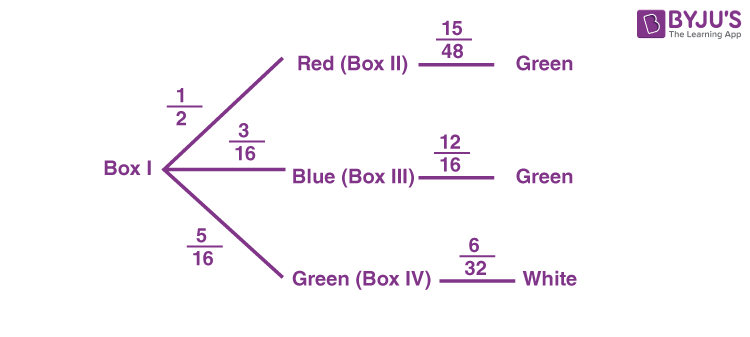Event A : One of the chosen ball is white

B : At least one of the chosen ball is green

$$\begin{array}{l}P\left( {\frac{A}{B}} \right) = \frac{{\frac{5}{{16}} \times \frac{6}{{32}}}}{{\frac{1}{2} \times \frac{{15}}{{48}} + \frac{3}{{16}} \times \frac{{12}}{{16}} + \frac{5}{{16}} \times 1}}\end{array}$$
$$\begin{array}{l} = \frac{5}{{52}}\end{array}$$

18. For positive integer n, define

$$\begin{array}{l}f(n) = n + \frac{{16 + 5n – 3{n^2}}}{{4n + 3{n^2}}} + \frac{{32 + n – 3{n^2}}}{{8n + 3{n^2}}} + \frac{{48 – 3n – 3{n^2}}}{{12n + 3{n^2}}} + … + \frac{{25n – 7{n^2}}}{{7{n^2}}}.\end{array}$$

Then, the value of

$$\begin{array}{l}\displaystyle \lim_{n \to \infty}f(n)\end{array}$$
is equal to

$$\begin{array}{l}(A)\ 3 + \frac{4}{3}{\log _e}7\end{array}$$
$$\begin{array}{l}(B)\ 4 – \frac{3}{4}{\log _e}\left( {\frac{7}{3}} \right)\end{array}$$
$$\begin{array}{l}(C)\ 4 – \frac{4}{3}{\log _e}\left( {\frac{7}{3}} \right)\end{array}$$
$$\begin{array}{l}(D) 3 + \frac{3}{4}{\log _e}7\end{array}$$

Sol.

$$\begin{array}{l}f(n) = n + \frac{{16 + 5n – 3{n^2}}}{{4n + 3{n^2}}} + \frac{{32 + n – 3{n^2}}}{{8n + 3{n^2}}} + ….. + \frac{{25n – 7{n^2}}}{{7{n^2}}}\end{array}$$
$$\begin{array}{l} = \left( {\frac{{16 + 5n – 3{n^2}}}{{4n + 3{n^2}}} + 1} \right) + \left( {\frac{{32 + n – 3{n^2}}}{{8n + 3{n^2}}} + 1} \right) + …….. + \left( {\frac{{25n – 7{n^2}}}{{7{n^2}}} + 1} \right)\end{array}$$
$$\begin{array}{l}f\left( n \right) = \frac{{9n + 16}}{{4n + 3{n^2}}} + \frac{{9n + 32}}{{8n + 3{n^2}}} + ….. + \frac{{25n}}{{7{n^2}}}\end{array}$$
$$\begin{array}{l} =\sum_{r = 1}^n {\frac{{9n + 16r}}{{4rn + 3{n^2}}}}=\frac{1}{n}\sum\limits_{r = 1}^n {\frac{{9 + 16\left( {\frac{r}{n}} \right)}}{{4\left( {\frac{r}{n}} \right) + 3}}} \end{array}$$
$$\begin{array}{l}\displaystyle \lim_{n \to \infty } f(n) = \int\limits_0^1 {\frac{{9 + 16x}}{{4x + 3}}dx} \end{array}$$
$$\begin{array}{l} = \int\limits_0^1 {\frac{{\left( {16x + 12} \right) – 3}}{{4x + 3}}dx} \end{array}$$
$$\begin{array}{l}\left. { = \left( {4x – \frac{3}{4}\;\ln \;\left| {4x + 3} \right|} \right)} \right|_0^1\end{array}$$
$$\begin{array}{l} = 4 – \frac{3}{4}\;\ln \;\frac{7}{3}\end{array}$$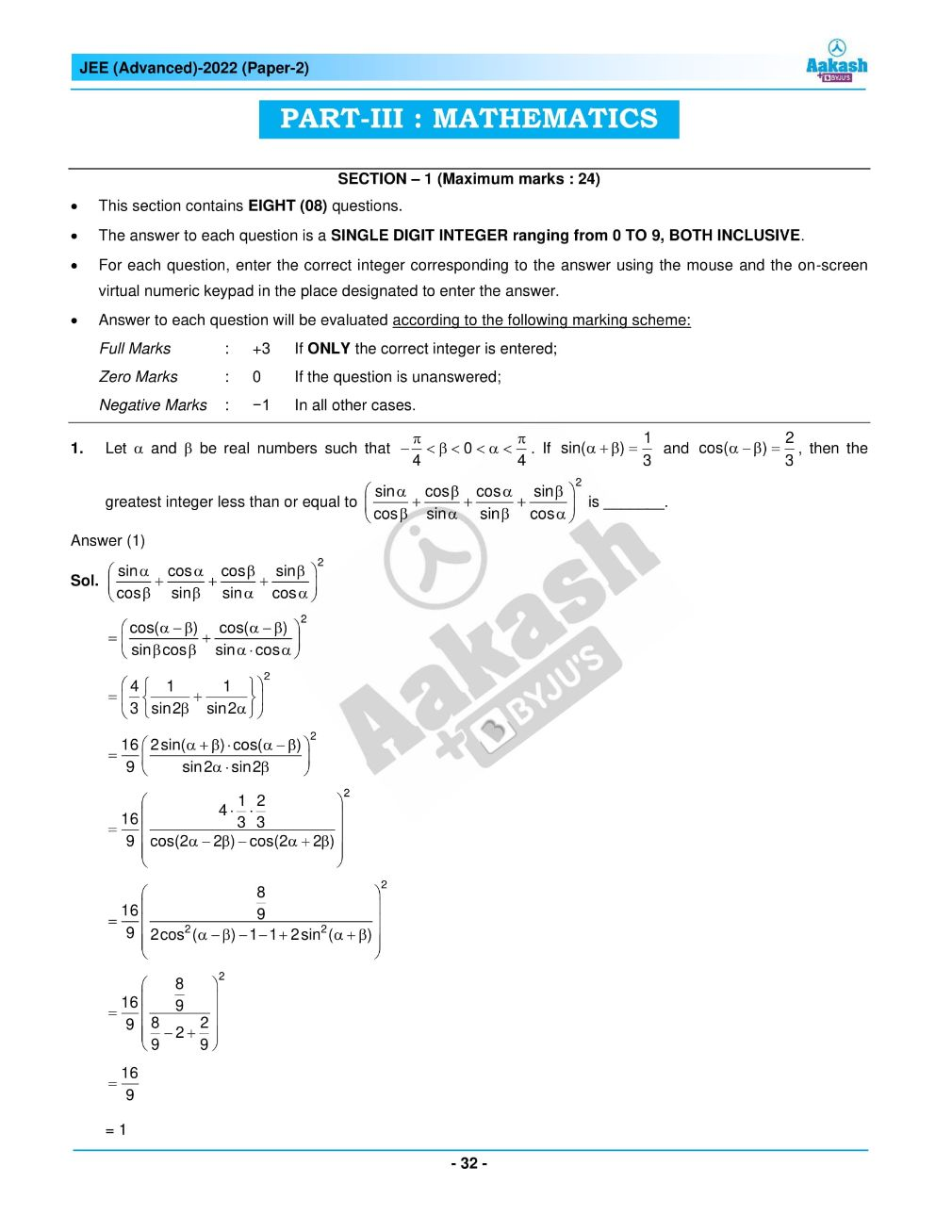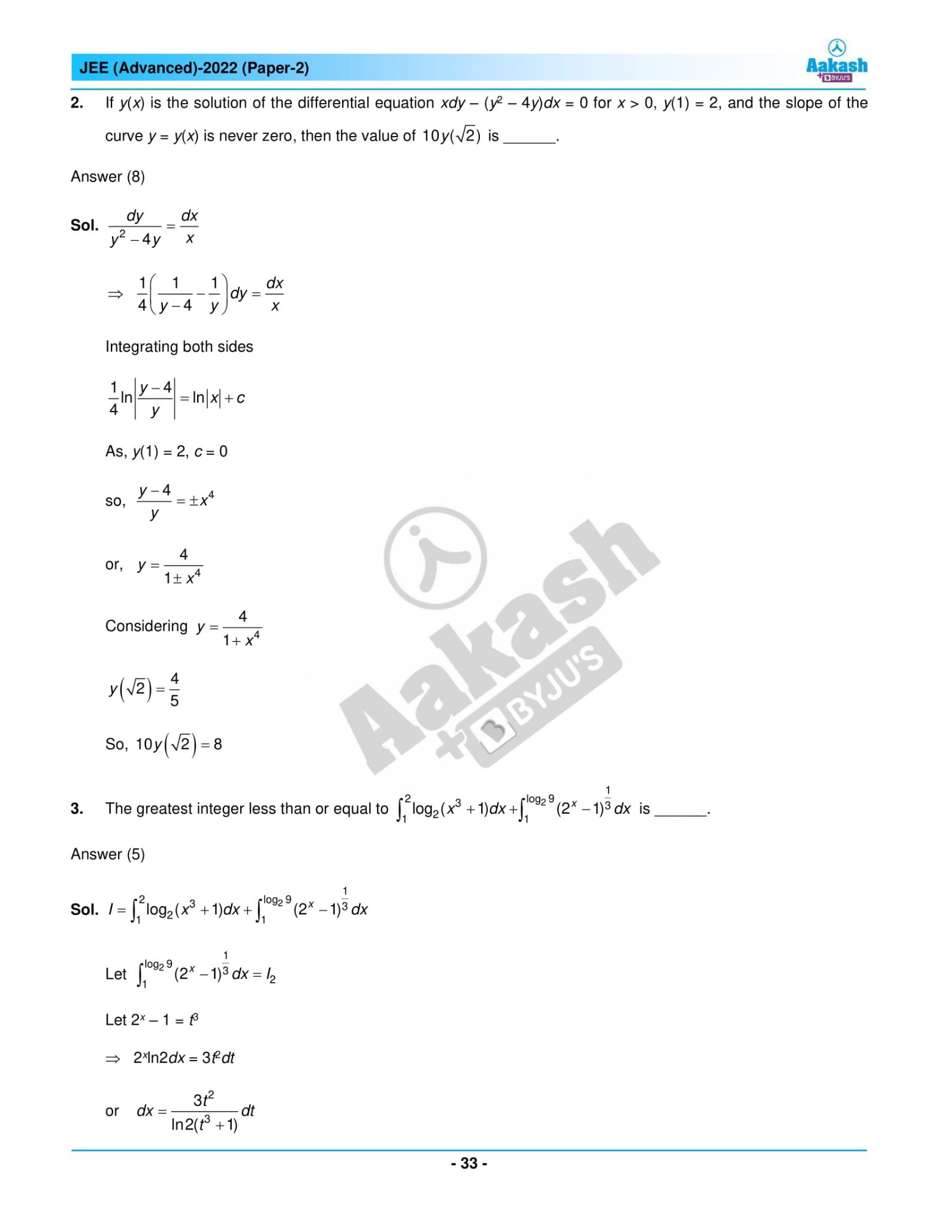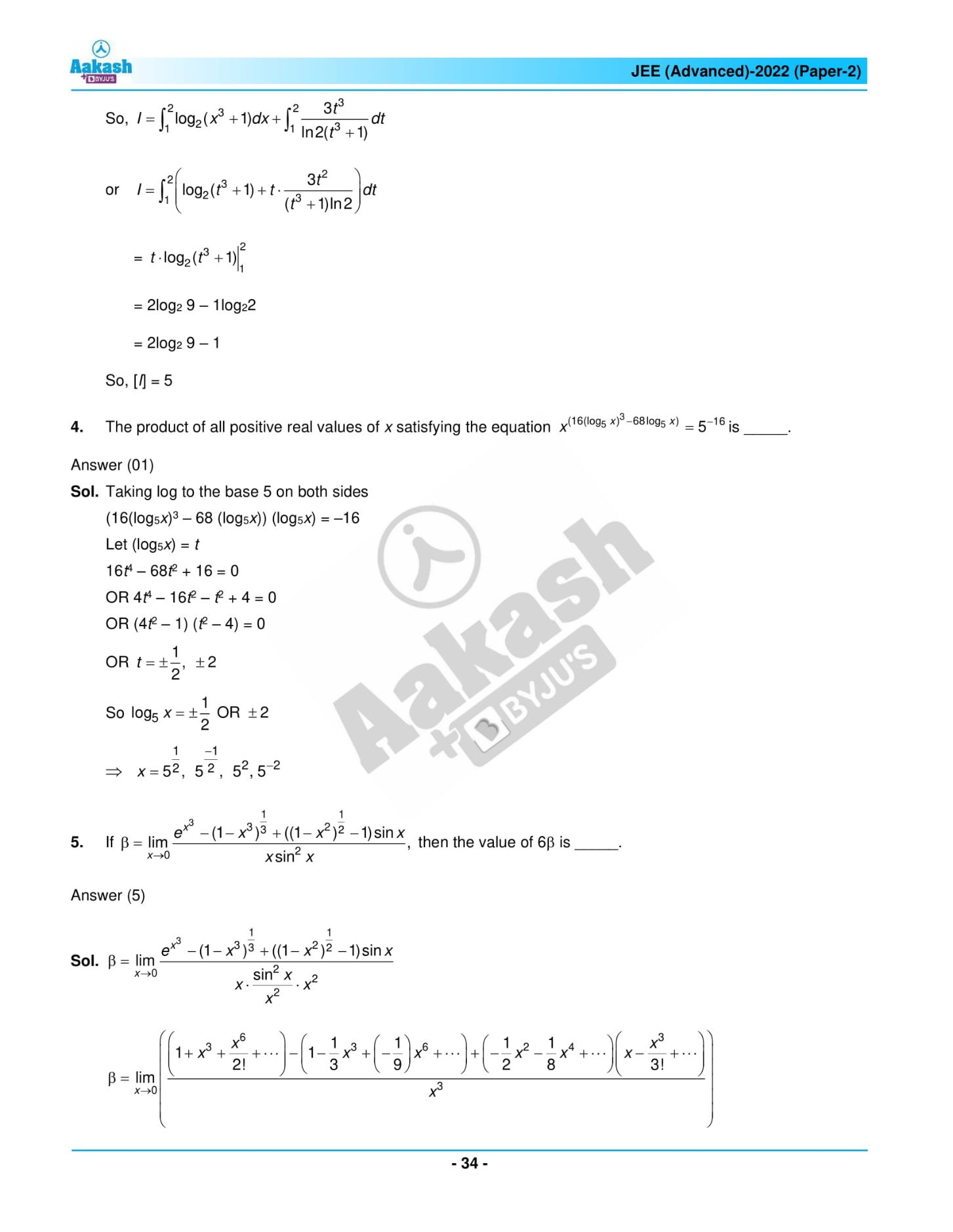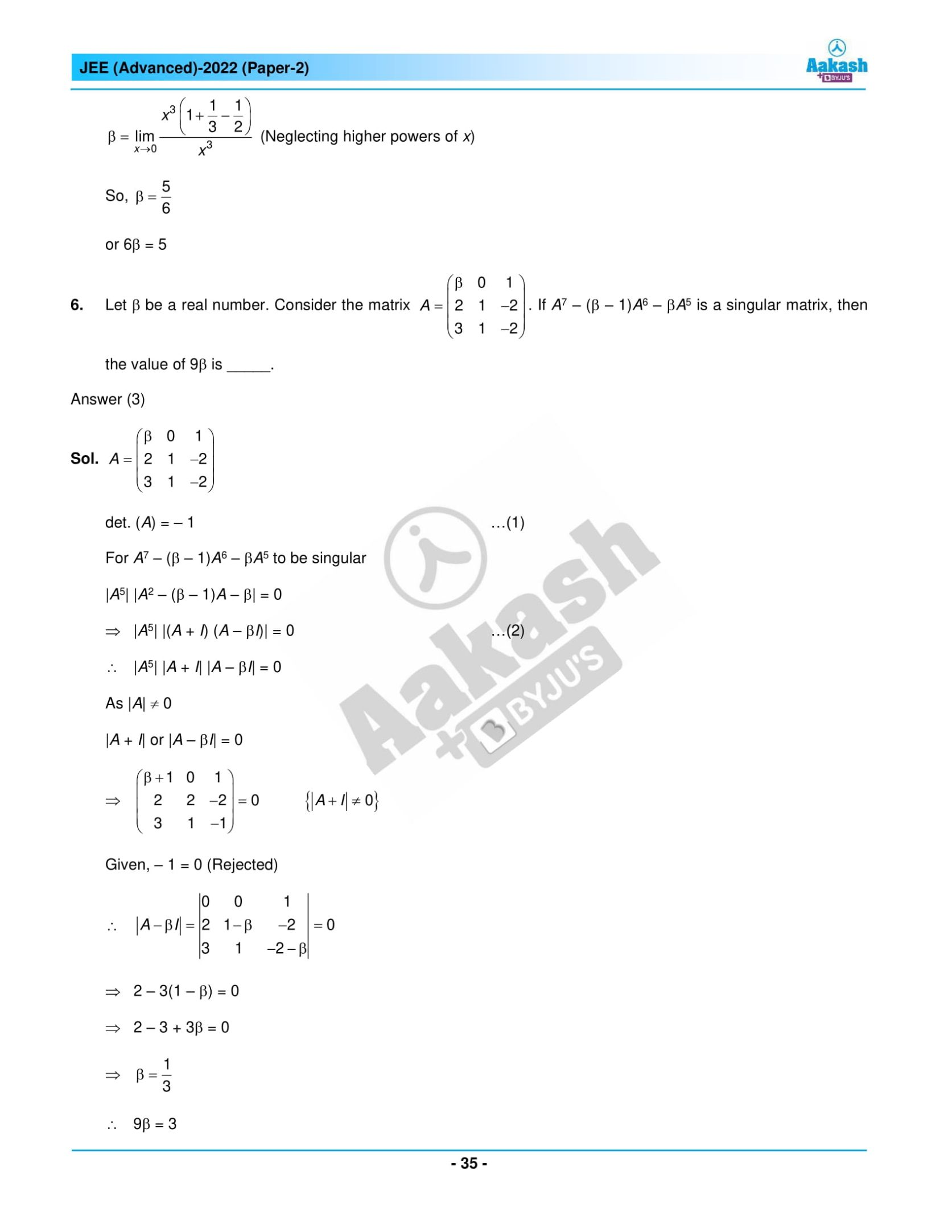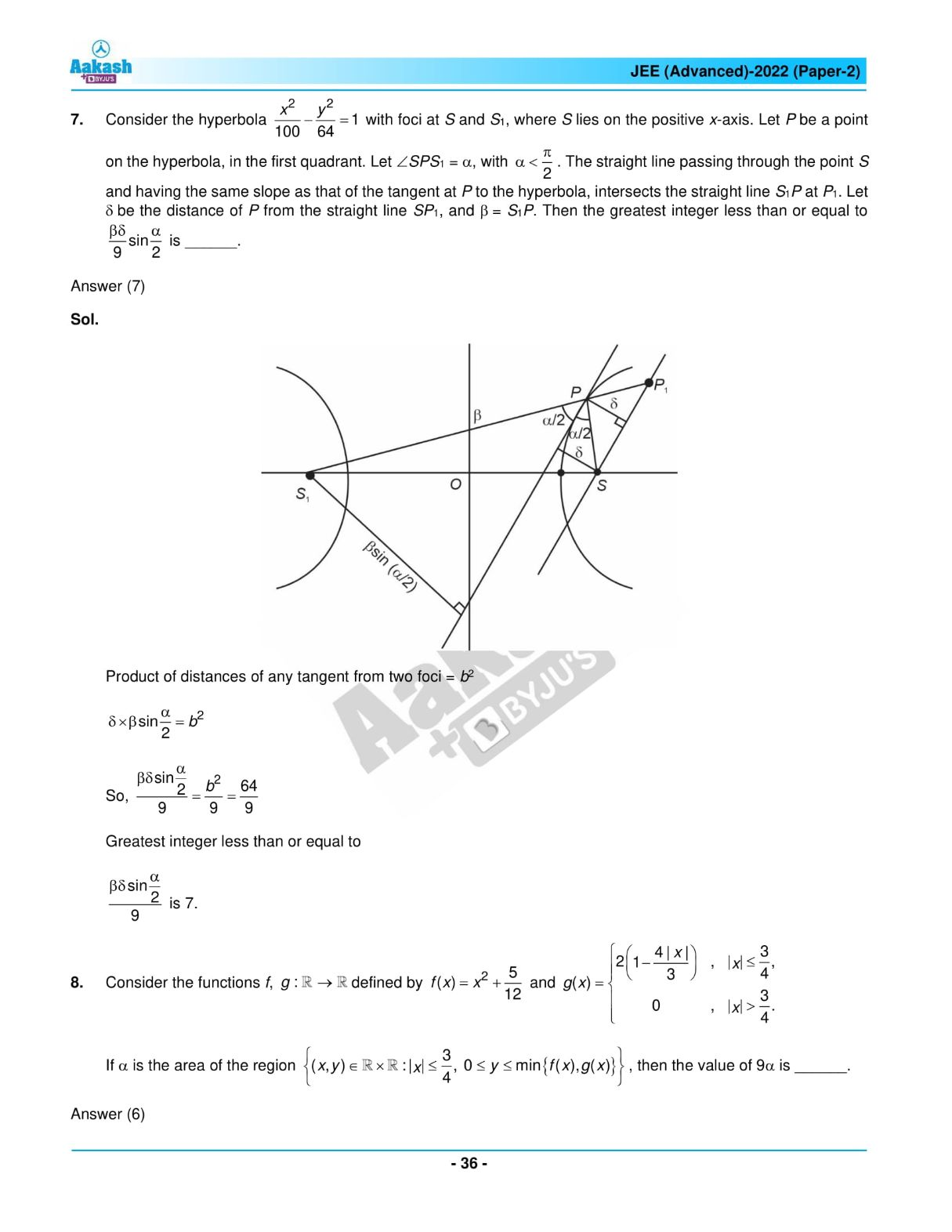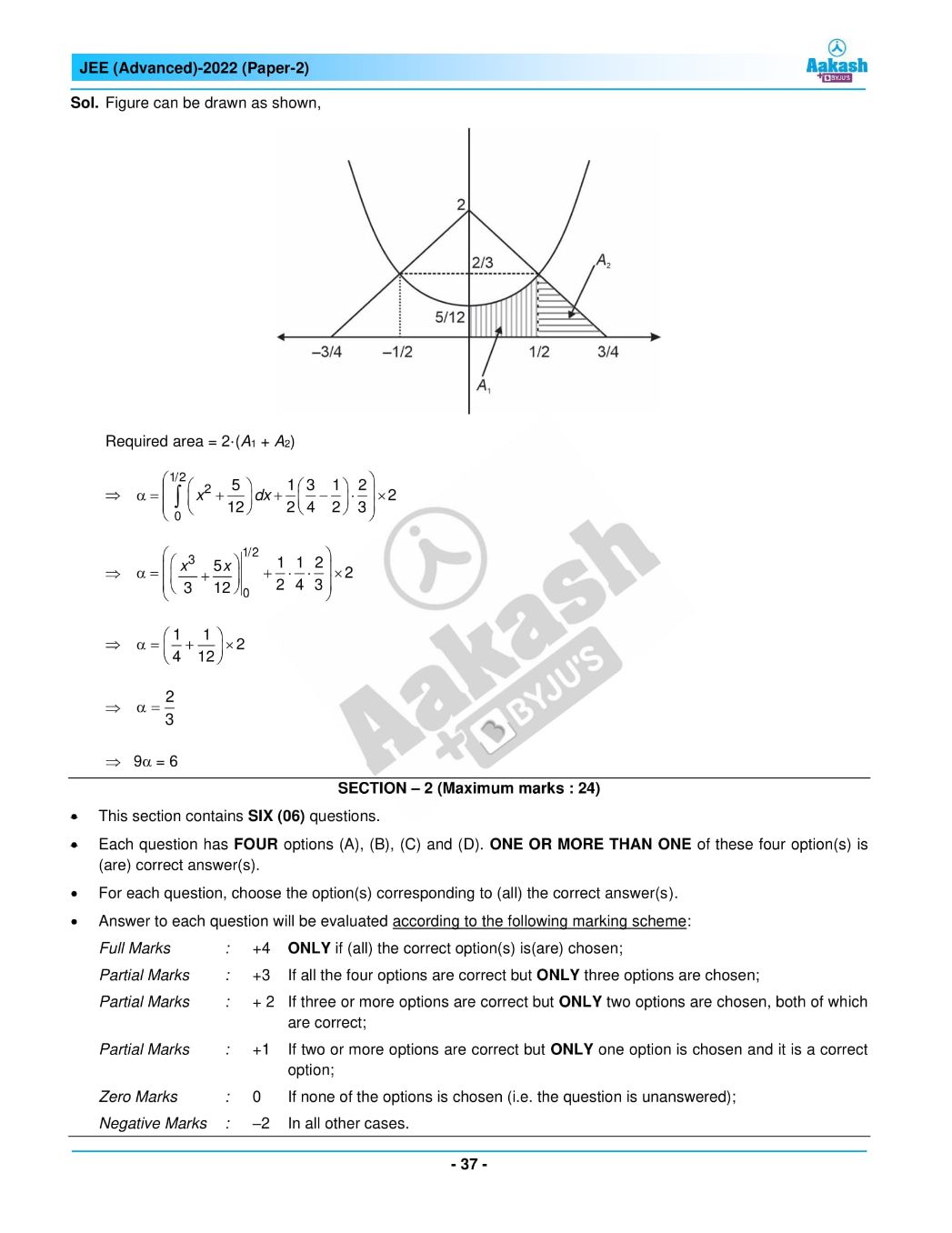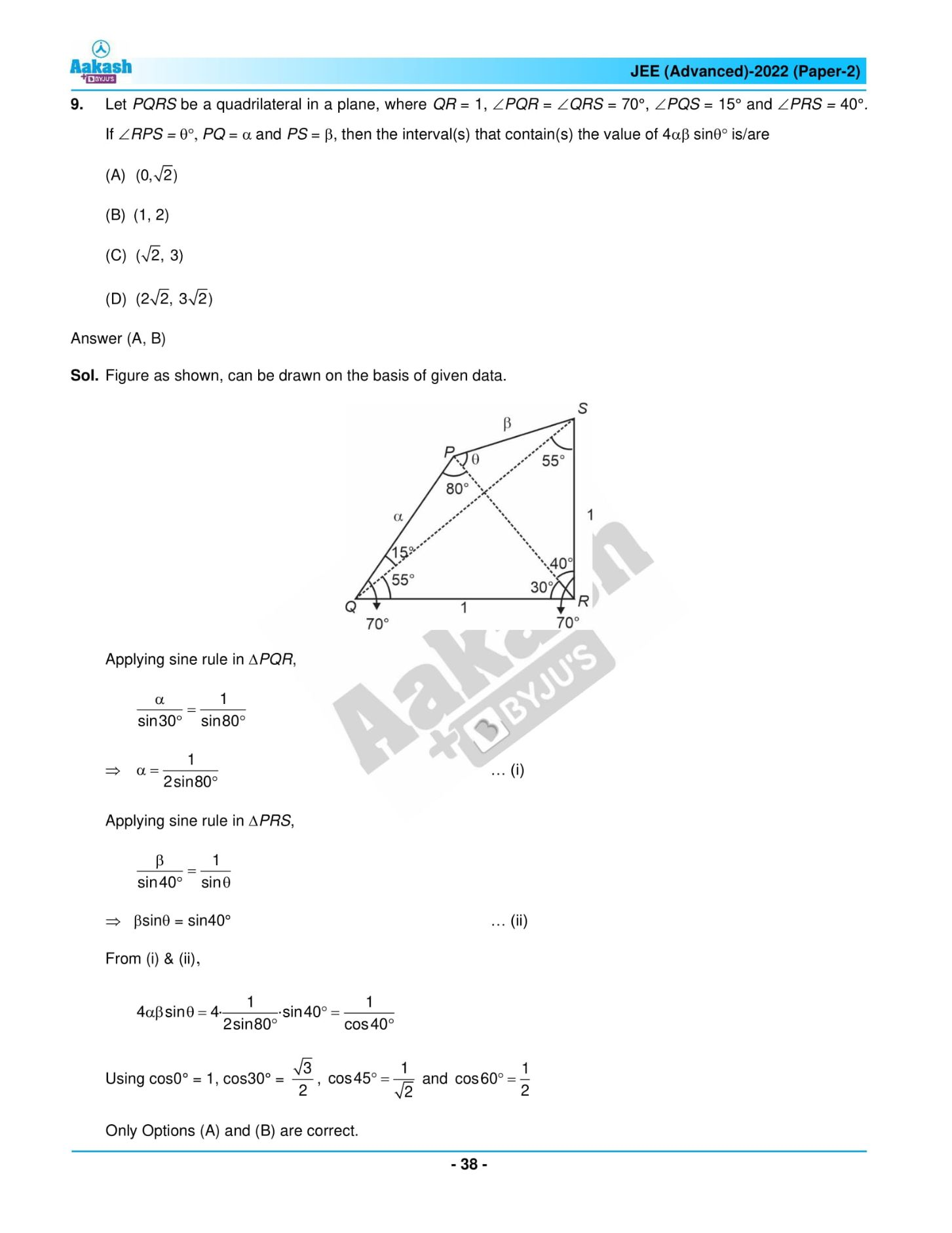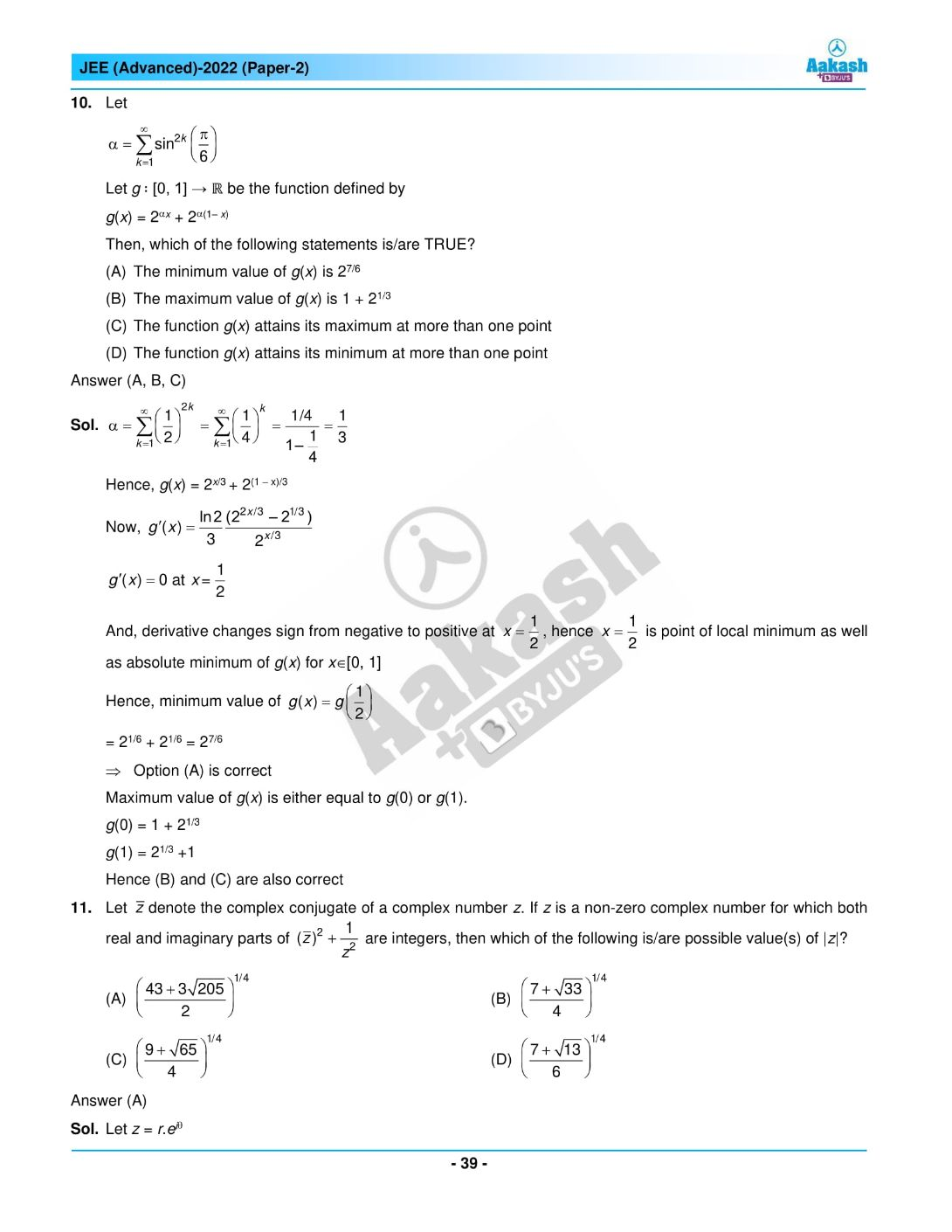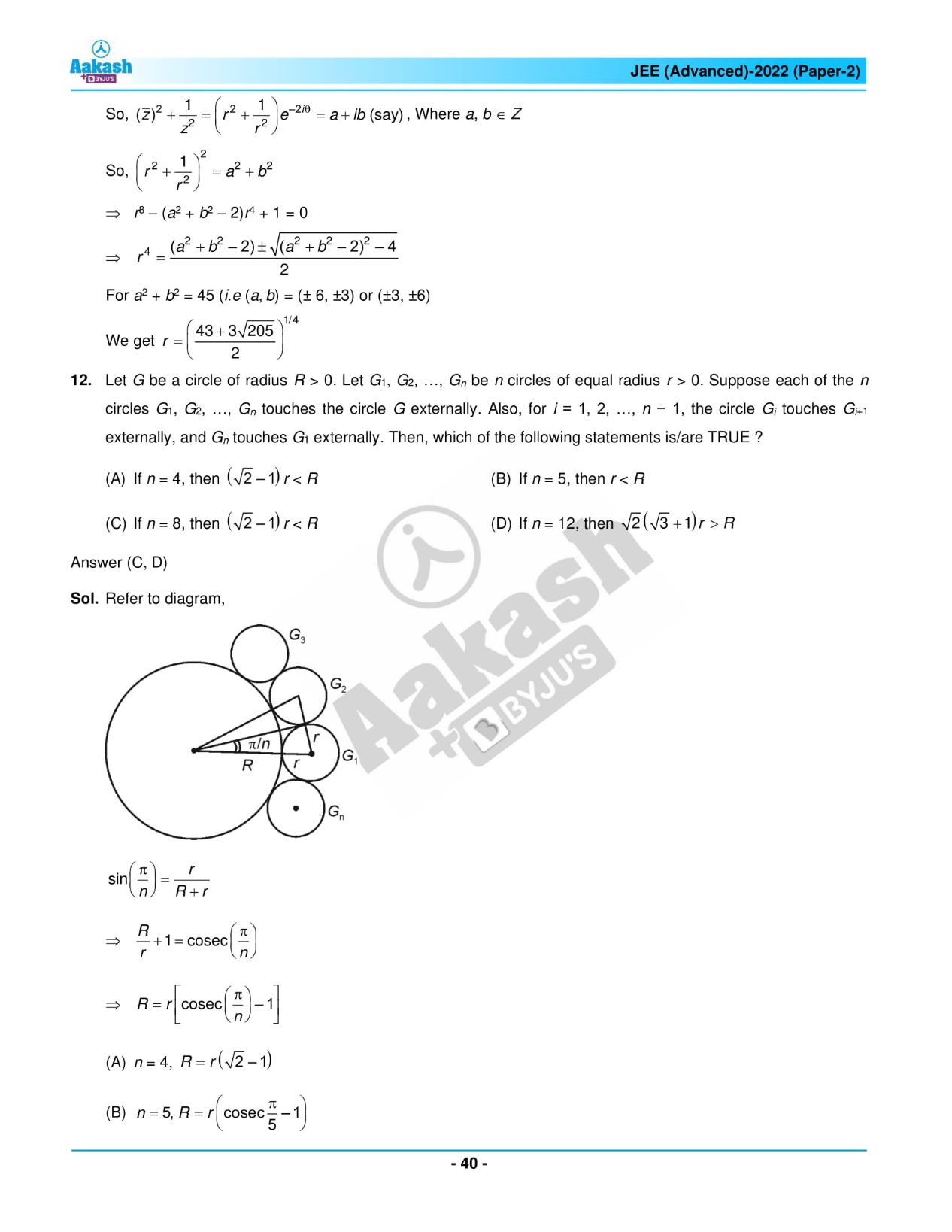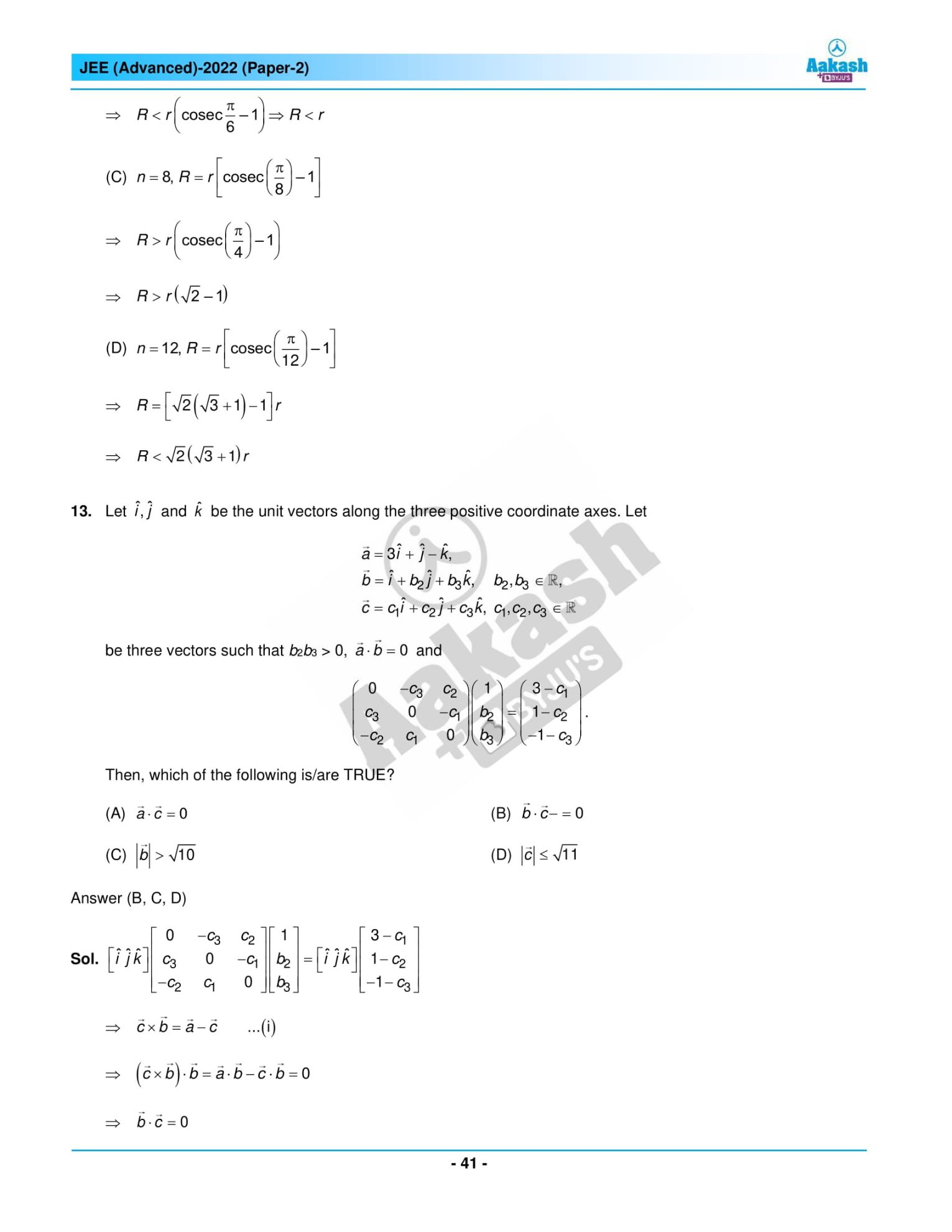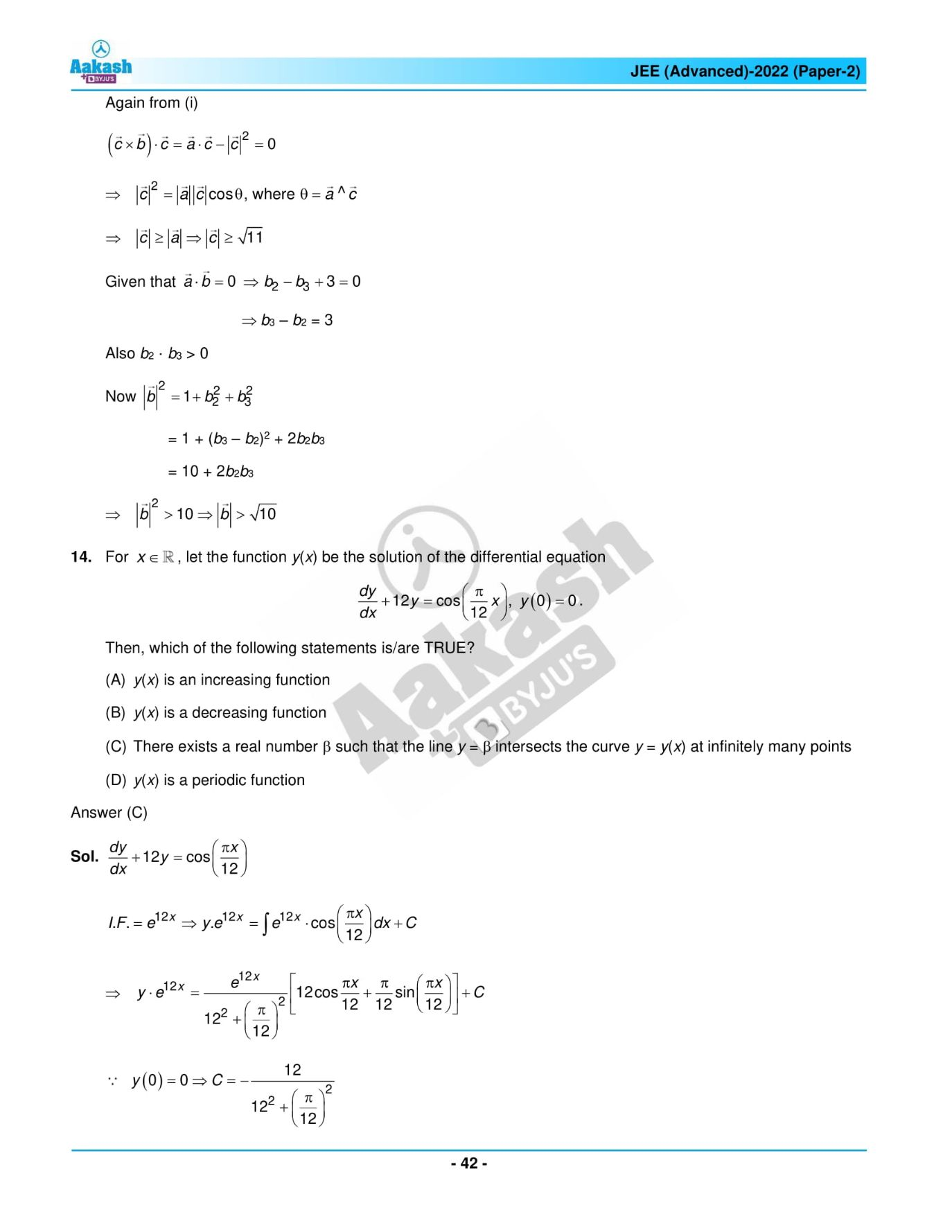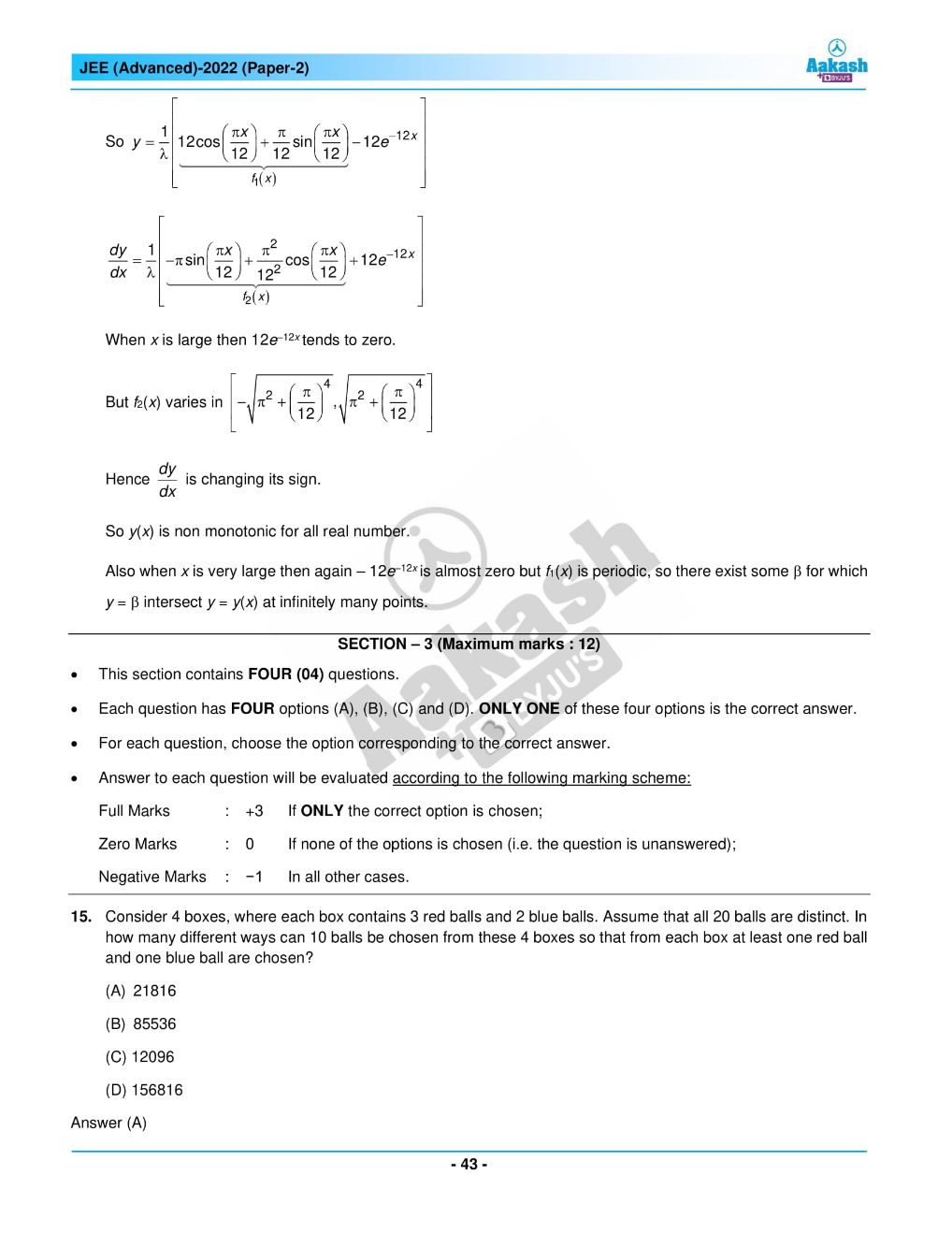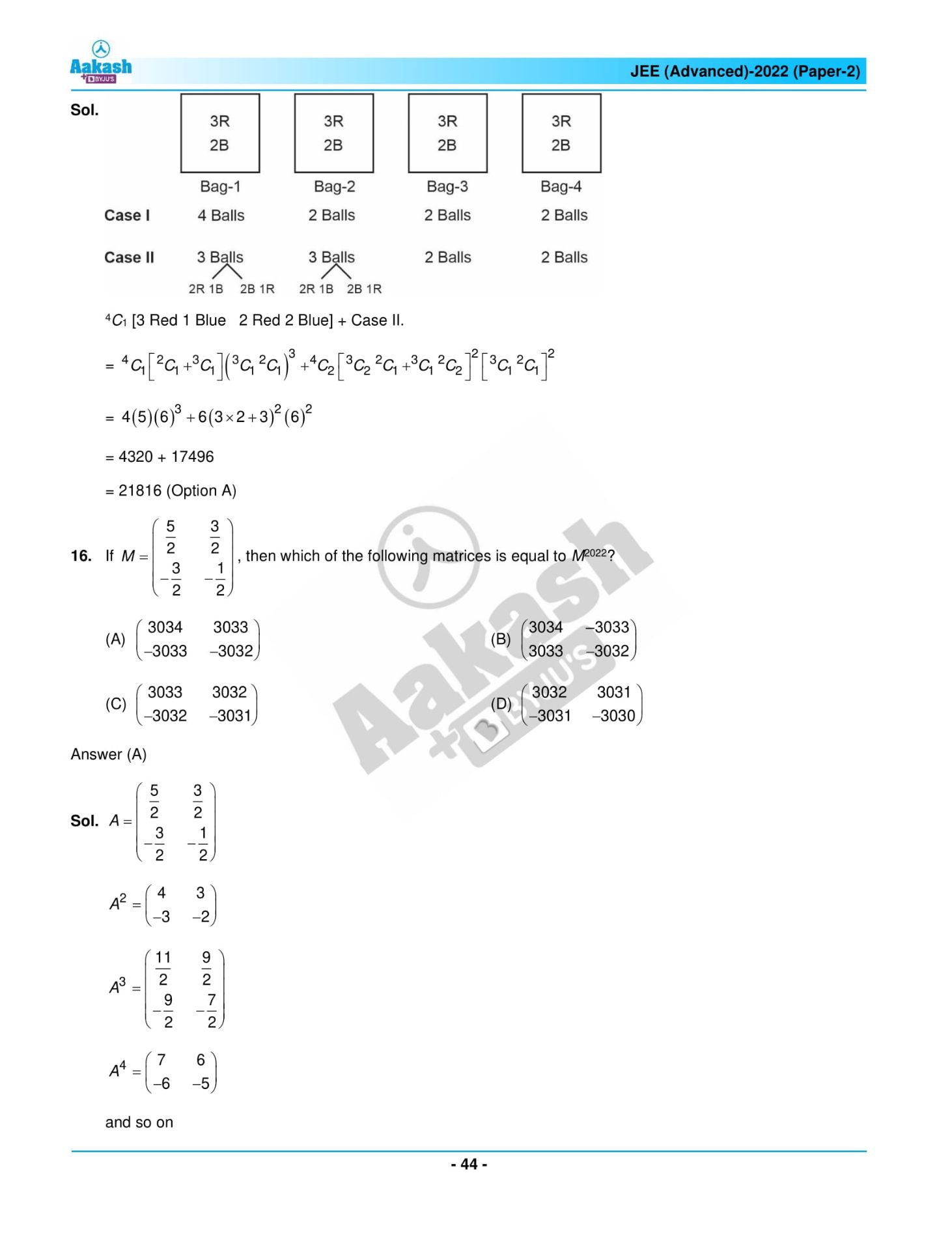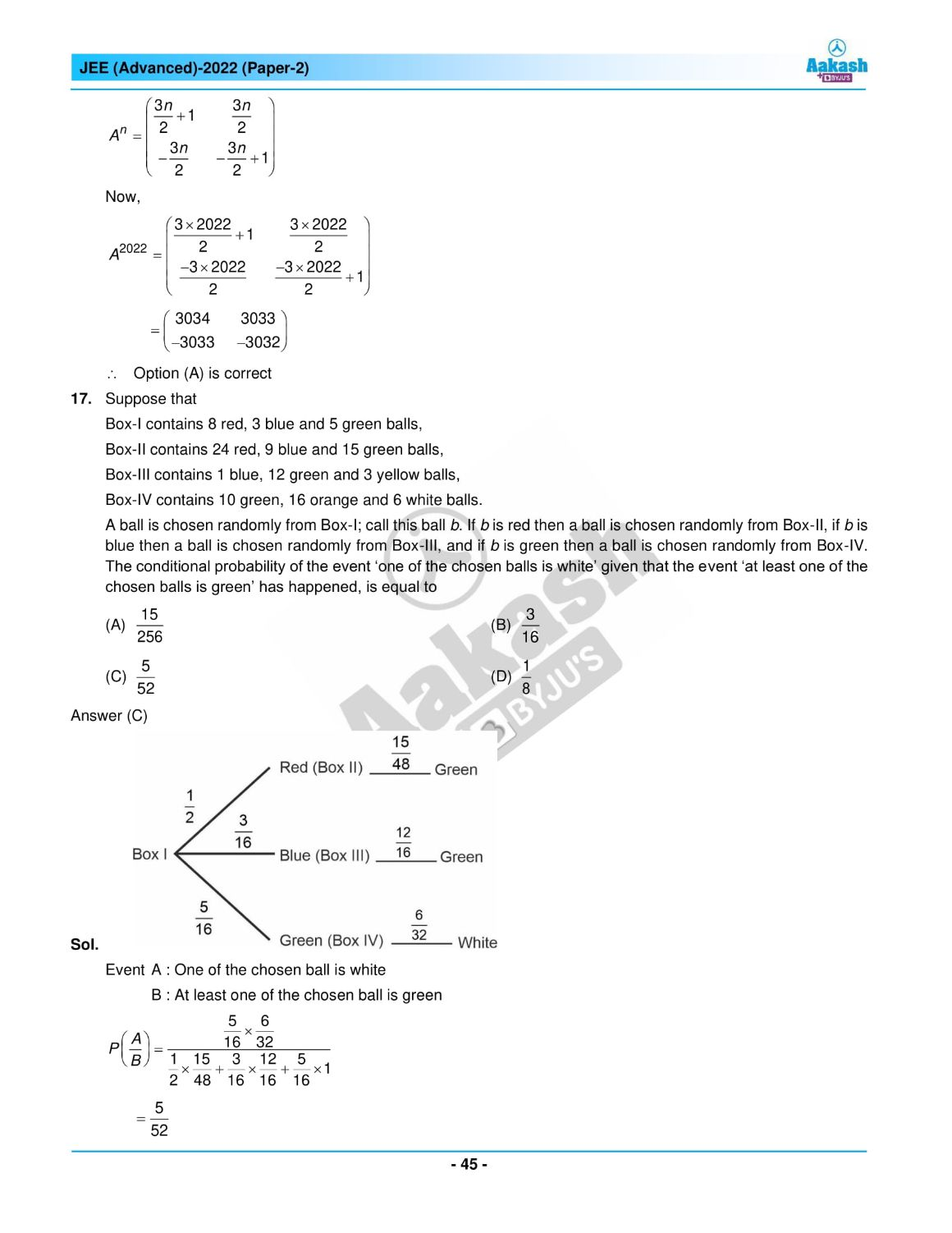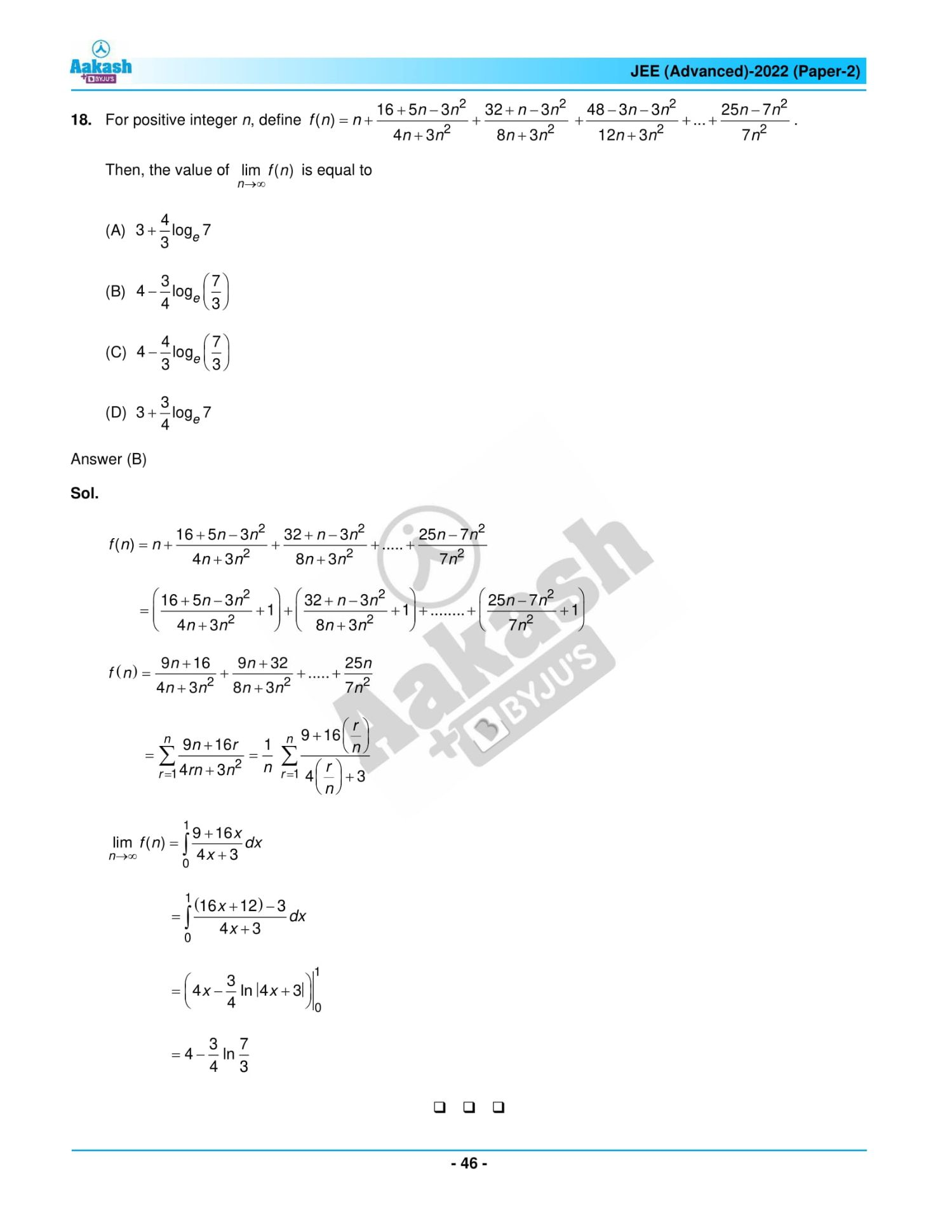## JEE Advanced 2022 Paper 2 – Live Analysis and Solutions## Frequently Asked Questions – FAQs

Q1

### What was the difficulty level of JEE Advanced 2022 Maths question paper 2?

Maths part had moderate to difficult level of questions to answer in the JEE Advanced 2022 question paper 2. However, we can determine the difficulty level of Maths paper 2 as 2.11 on a scale of 3.
Q2

### Were there any questions from Probability in JEE Advanced question paper 2022 Maths paper 2?

Yes, one question asked from the Probability chapter and is based on the Bayes theorem.
Q3

### How many marks can be awarded for every correct MCQ in JEE Advanced 2022 Maths paper 2?

3 marks will be awarded for every correct MCQ in JEE Advanced 2022 Maths paper 2.
Q4

### How many tough questions appeared in the JEE Advanced 2022 Mathematics paper 2?

6 tough questions appeared in the JEE Advanced 2022 Mathematics paper 2.
Q5

### How many questions were asked from the chapter Differential equations in the Maths part of JEE Advanced question paper 2022, paper 2?

Two questions were asked from the chapter Differential equations in the Maths part of JEE Advanced question paper 2022, paper 2.